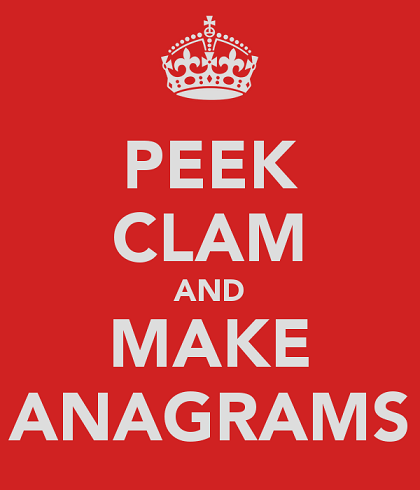# Ronaldo, Arnoldo and OrnaldoSometimes,two or more people may have names that are anagrams of each other. Let us call a group of distinct names that are all anagrams of each other an anagramic tuple.

For example (Norma, Ramon, Roman) is an example of an anagramic tuple of size $3$.

In names.txt, a 46K text file containing over five-thousand first names, let the size of the largest anagramic tuple be $N$. Let $M$ be the number of anagramic tuples of size $N$. What is the value of the product $N \times M$?

Details and assumption

• The arrangement of the names in the tuple doesn't matter. I.e. (Tia, Tai) is the same tuple as the tuple (Tai, Tia).

• All the names in an anagramic tuple are distinct.

×